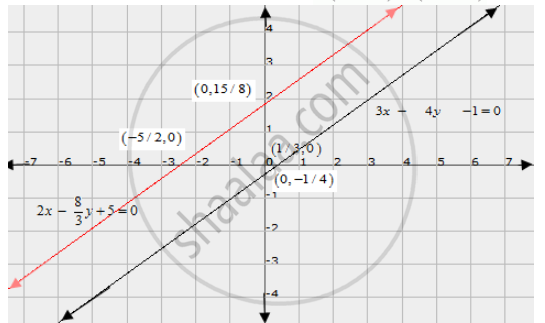# Show Graphically that Each One of the Following Systems of Equations is Inconsistent (I.E. Has No Solution) : 3x − 4y − 1 = 0 2x - 8/3y + 5 = 0 - Mathematics

Show graphically that each one of the following systems of equations is inconsistent (i.e. has no solution) :

3x − 4y − 1 = 0

2x - 8/3y + 5 = 0

#### Solution

The given equations are

3x - 4y - 1 = 0 .....(i)

2x - 8/3 y + 5 = 0

6x - 8y + 15 = 0 ...(ii)

Putting x = 0 in equation (i) we get

 => 3 xx 0 - 4y = 1

=> y = -1/4

=> x = 0, y = -1/4

Putting y = 0 in equation (i) we get

=> 3x - 4xx0 = 1

=> x = 1/2

=> x = 1/3, y = 0

Use the following table to draw the graph.

 x 0 1/3 y -1/4 0

The graph of (i) can be obtained by plotting the two points A(0, -1/4), B(1/3, 0)

6x - 8y = -15  ....(ii)

Putting x = 0 in equation (ii) we get

=> 6 xx 0 - 8y = -15

=> y = 15/8

=> x = 0, y = 15/8

Putting y = 0 in equation (ii) we get

=> 6x - 8 xx 0 = -15

=> x = -15/6

=> x = -5/2, y = 0

Use the following table to draw the graph.

 x 0 -5/2 y 15/8 0

Draw the graph by plotting the two point C(0,15/8), D(-5/2, 0) from tableHere, the two lines are parallel.

Hence the given system of equations is inconsistent.

Concept: Graphical Method of Solution of a Pair of Linear Equations
Is there an error in this question or solution?

#### APPEARS IN

RD Sharma Class 10 Maths
Chapter 3 Pair of Linear Equations in Two Variables
Exercise 3.2 | Q 18 | Page 29